# De-Moivre's Theorem

Go back to  'Complex Numbers'

DE - MOIVRE’S THEOREM

Let $$z = r{e^{i\theta }}$$

\begin{align}{} {\bf{(a)}}\quad\text{ If } n\text{ is an integer},\; {z^n} &= {(r{e^{i\theta }})^n} = {r^n}{e^{in\theta }}\\ \,\,\,\,\,\,\,\,\,\,\,\,\,\,\,\,\,\,\,\,\,\,\,\,\, &= {r^n}(\cos n\theta + i\sin n\theta ) \end{align}

\begin{align}{\bf{(b)}}\quad\text{If } n \text{ is a non-integer rational number, say of the form }\frac{p}{q},\end{align}

\begin{align}&{z^n} = {(r{e^{i\theta }})^{\frac{p}{q}}}\\\,\,\,\,\, &\quad= {r^{p/q}}{e^{\frac{{ip\theta }}{q}}} = {r^{p/q}}(\cos \frac{{p\theta }}{q} + i\sin \frac{{p\theta }}{q})\end{align}

is one of the values of $${z^n}$$ . There will be actually multiple values of $${z^n}$$ . (How to obtain those multiple values will be discussed subsequently).

We will now discus show to evaluate the $${n^{th}}$$ roots of an arbitrary complex number in the manner described above.

\begin{align}&\;\;\;Let\,\,\,z = r{e^{i(2p\pi + \theta )}}\\ &\Rightarrow \,\,\,{z^{\frac{1}{n}}} = {\{ r{e^{i(2p\pi + \theta )}}\} ^{\frac{1}{n}}}\\\,\,\,\,\,\,\,\,\,\,\,\,\, &\qquad\quad\;= {r^{1/n}}{e^{i\left( {\frac{{2p\pi + \theta }}{n}} \right)}}\end{align}

This will have $$n$$ unique values given by $$n$$ successive integral values of $$p.$$ We take $$p$$ from the set $${0, 1, 2...(n–1)}.$$

The $$n$$ values that we obtain are listed out below:

\begin{align}&{r^{1/n}}{e^{\frac{{i\theta }}{n}}},\,\,{r^{1/n}}{e^{i\left( {\frac{{2\pi }}{n} + \frac{\theta }{n}} \right)}},\,\,{r^{1/n}}{e^{i\left( {\frac{{4\pi }}{n} + \frac{\theta }{n}} \right)}}...,\,{r^{1/n}}{e^{i\left( {\frac{{2p\pi }}{n} + \frac{\theta }{n}} \right)}}...{r^{1/n}}{e^{i\left( {\frac{{2(n - 1)\pi }}{n} + \frac{\theta }{n}} \right)}}\end{align}

These $$n$$ values of $${z^{1/n}}$$ are termed the $${n^{th}}$$ roots of $$z.$$ How will they lie on a plane? Notice that the angle between any two successive roots \begin{align}{z_i}\,{\rm{ and }}\,{z_{i + 1}}{\rm{ is }}\frac{{2\pi }}{n}.\end{align} Thus, the $${n^{th}}$$ roots will lie on a circle of radius $${r^{1/n}}$$ and will be “evenly spaced out”; the angle between any two successive roots being \begin{align}\frac{{2\pi }}{n}\end{align}.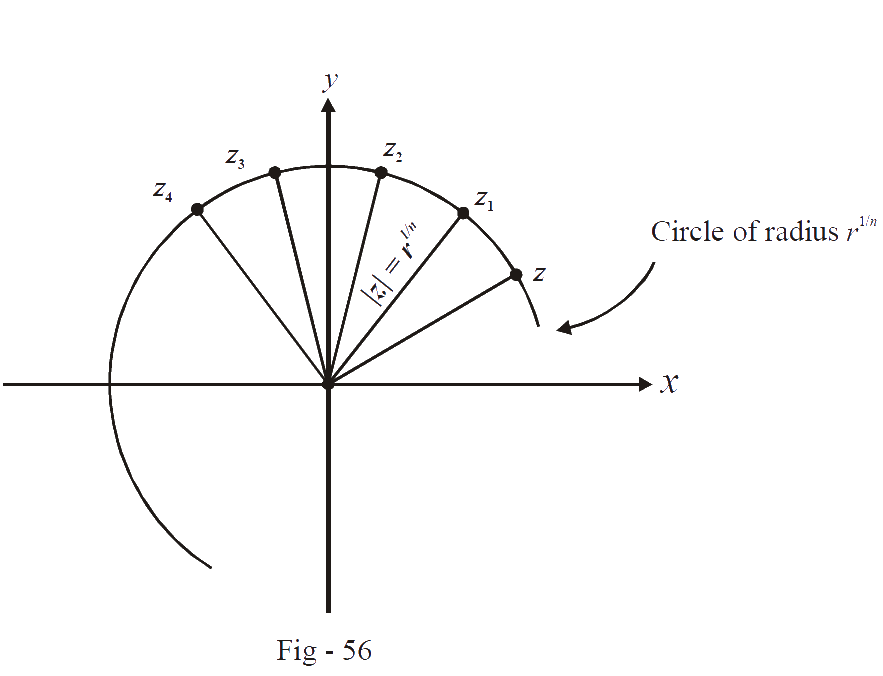Now we consider a special case, the $${n^{th}}$$ roots of unity (1). In other words, we want the solutions to the equation:

${z^n} = 1$

The Euler’s form of 1 is $${e^{i0}}.$$ Therefore,

\begin{align}&z = {e^{i\frac{{(2p\pi + 0)}}{n}}}\\\,\,\, &\;= {e^{\frac{{i2p\pi }}{n}}}\end{align}

The $$n$$ different values are

\begin{align}&1,\,\,{e^{\frac{{i2\pi }}{n}}},\,\,{e^{\frac{{i4\pi }}{n}}}...,\,\,{e^{\frac{{i2(n - 1)\pi }}{n}}}\end{align}

If we let $${e^{i2\pi /n}} = \alpha$$ , the n root are $$1,\,\,\alpha ,\,\,{\alpha ^2}...\,\,{\alpha ^{n - 1}}$$

These $$n$$ roots will be evenly spaced out, lying on a circle of radius 1 centred at the origin..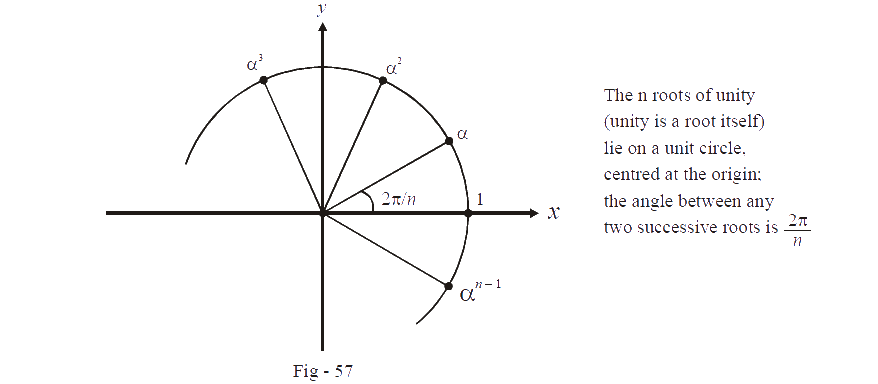From symmetry, notice that the sum of these $$n$$ roots (vectors) will be 0.

$1 + \alpha + {\alpha ^2} + ... + {\alpha ^{n - 1}} = 0$

This can be proved analytically too:

Since $$\alpha$$ is one of the $${n^{th}}$$ roots of 1, we have

\begin{align}&\quad\quad\;{\alpha ^n} = 1\\ &\Rightarrow \,\,\,{\alpha ^n} - 1 = 0\\ &\Rightarrow \,\,\,(\alpha - 1)({\alpha ^{n - 1}} + {\alpha ^{n - 2}} + ... + \alpha + 1) = 0\end{align}

Since $$\alpha \ne 1$$ , we get:

${\alpha ^{n - 1}} + {\alpha ^{n - 2}} + ... + \alpha + 1 = 0$

Now we evaluate the product of these n roots:

\begin{align}&P = 1 \cdot \alpha \cdot {\alpha ^2}...{\alpha ^{n - 1}}\\ &\;\;= {\alpha ^{1 + 2 + 3 + ... + n - 1}}\\ &\;\;= {\alpha ^{\frac{{n(n - 1)}}{2}}}\end{align}

Since \begin{align}&\alpha = {e^{i2\pi /n}},P = {e^{\frac{{i2\pi }}{n} \cdot \left( {\frac{{n(n - 1)}}{2}} \right)}} = {e^{i\pi (n - 1)}}\end{align} .

Now observe that if $$n$$ is odd, $$P$$ is 1 while if $$n$$ is even, $$P$$ is –1:

$P = \,\,\left\{ {\begin{array}{*{20}{c}}1&{{\rm{if \,\,}}n{\rm{ \,\,is \,\,odd}}}\\{ - 1}&{{\rm{if \,\,}}n{\rm{ \,\,is \,\,even}}}\end{array}} \right\}$

Lets apply this discussion to some particular values of $$n:$$

(i)   $$\fbox{n = 2}$$

We want the square roots of unity, or, the solutions to

${z^2} = 1$

One of the roots is 1. Where can we symmetrically place the other root? Obviously, at –1.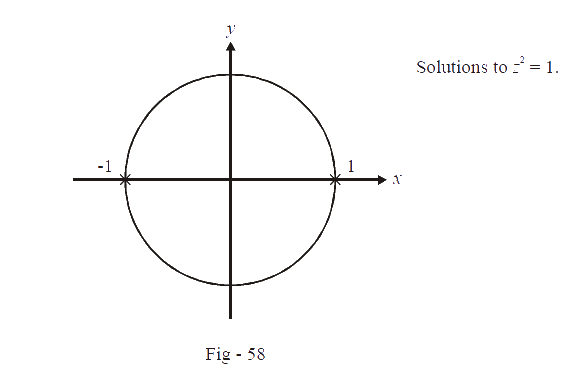(ii) $$\fbox{n = 3}$$

We want to solve

${z^3} = 1$

One of the roots is 1. The other roots can be placed symmetrically if they are at angles, \begin{align}&\frac{{2\pi }}{3}{\rm{ and }}\frac{{4\pi }}{3}\end{align} as shown below: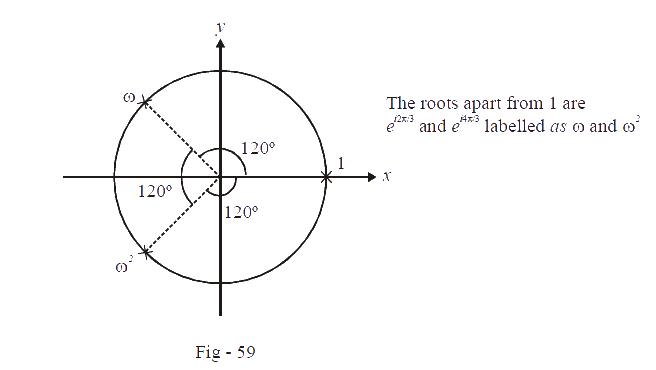The two other roots are:

$\omega = {e^{i\,2\pi /3}} = - \frac{1}{2} + \frac{{i\sqrt 3 }}{2},\,\,{\omega ^2} = {e^{i\,4\pi /3}} = - \frac{1}{2} - \frac{{i\sqrt 3 }}{2}$

Notice that $$w\,\,and\,\,w{^2}$$  are conjugates of each other. Also, $$1 + \omega + {\omega ^2} = 0$$ and $$1 \cdot \omega \cdot {\omega ^2} = {\omega ^3} = 1$$

(iii) $$\fbox{n = 4}$$

We want to solve

${z^4} = 1$

The four roots can be symmetrically placed as shown below: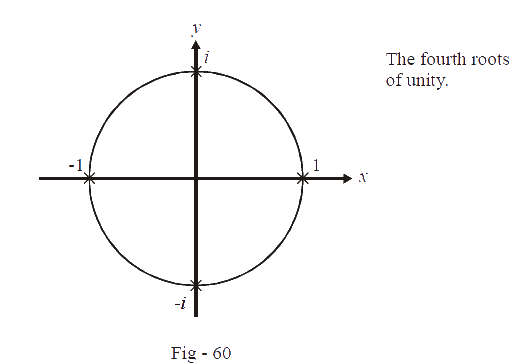Note that $$1 + i + ( - 1) + ( - i) = 0\,\,{\rm{and }}1 \cdot (i) \cdot ( - 1) \cdot ( - i) = - 1$$ .

Higher order roots can be similarly evaluated. You must understand carefully the geometrically significance of the $${n^{th}}$$ roots. Let us take one of the cube roots of unity for this purpose, say $${\omega ^2}$$ .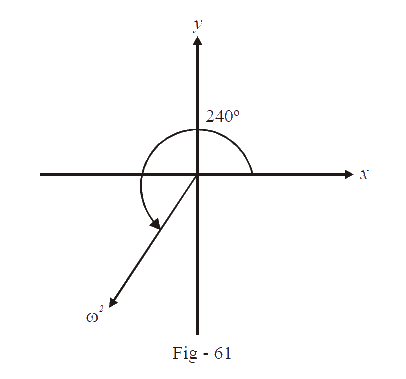If you cube $${\omega ^2}$$ , you are essentially rotating this vector. The argument of $${\omega ^2}$$  is 240° ; when you cube $${\omega ^2}$$ , you will rotate  by 240°  $$({\rm{for\,\, }}{\omega ^2} \times {\omega ^2}) + {\rm{another\,\,}} 240^\circ ({\rm{for\,\, }}{\omega ^2} \times {\omega ^2} \times {\omega ^2})$$ .  Hence, $${\omega ^2}$$ , when cubed, will become the vector 1.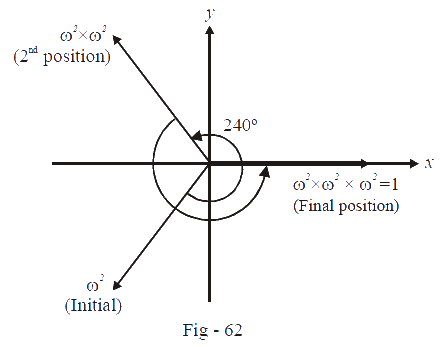Thus,

${({\omega ^2})^3} = 1$

Similarly, observe one of the fourth roots of unity, say $$i.$$ The argument of $$i$$ is 90°. When you raise $$i$$ to power 4, you are essentially rotating the vector $$i$$ by 90°(for$$i × i$$) + 90°(for $$i ×i ×i$$) + 90°(for $$i×i×i×i$$). Therefore, $$i$$ when raised to power four will become the vector 1.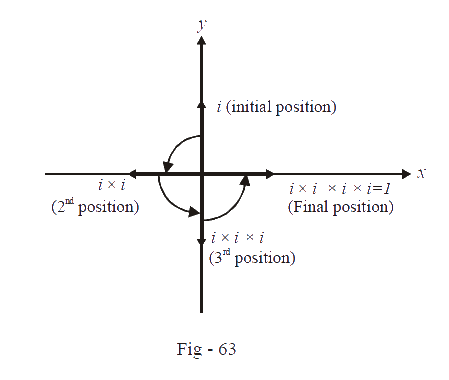Thus,

${i^4} = 1$

This discussion should make you realise that when looking for the $${n^{th}}$$ roots of unity, you are looking for vectors which when rotated by a certain fixed angle $$(2\pi /n)$$  a particular number $$(n – 1)$$ of times, give the vector 1.

Learn from the best math teachers and top your exams

• Live one on one classroom and doubt clearing
• Practice worksheets in and after class for conceptual clarity
• Personalized curriculum to keep up with school